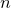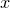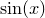## 6.5.3 Plotting data from vectors

Vectors can be used to pass calculated data to the plot command for plotting. Instead of supplying the name of a data file, or a function to be plotted, a series of colon-separated vector objects should be passed to the plot command. Each of the vectors should be the same length; theth elements of each of the vectors are put together to form the columns of data for theth data point.

The following example draws 100 random points on a graph:

N=100
a=vector(N) ; b=vector(N)
for i=0 to 99 { a[i]=random.random() ; }
for i=0 to 99 { b[i]=random.random() ; }
plot [0:1][0:1] a:b


Vectors support the same filter(), map() and reduce() methods as lists (see Section 6.3.6), and these can prove especially useful for preparing data for plotting. The following example selects fifty random points along the-axis, and uses them to plot:

N=50
a=vector(N)
for i=0 to 99 { a[i]=random.random() ; }
b=a.map(sin)
plot [0:1][0:1] a:b